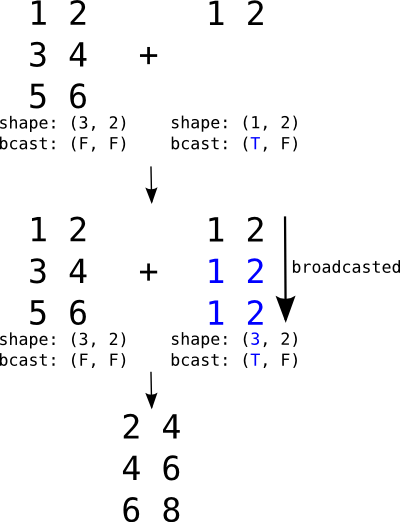Broadcasting is a mechanism which allows tensors with different numbers of dimensions to be added or multiplied together by (virtually) replicating the smaller tensor along the dimensions that it is lacking.

Broadcasting is the mechanism by which a scalar may be added to a matrix, a vector to a matrix or a scalar to a vector.Broadcasting a row matrix. T and F respectively stand for True and False and indicate along which dimensions we allow broadcasting.

If the second argument were a vector, its shape would be `(2,)` and its broadcastable pattern `(False,)`. They would be automatically expanded to the left to match the dimensions of the matrix (adding `1` to the shape and `True` to the pattern), resulting in `(1, 2)` and `(True, False)`. It would then behave just like the example above.

Unlike numpy which does broadcasting dynamically, Aesara needs to know, for any operation which supports broadcasting, which dimensions will need to be broadcasted. When applicable, this information is given in the Type of a Variable.

The following code illustrates how rows and columns are broadcasted in order to perform an addition operation with a matrix:

```>>> r = at.row()
(True, False)
>>> mtr = at.matrix()
(False, False)
>>> f_row = aesara.function([r, mtr], [r + mtr])
>>> R = np.arange(3).reshape(1, 3)
>>> R
array([[0, 1, 2]])
>>> M = np.arange(9).reshape(3, 3)
>>> M
array([[0, 1, 2],
[3, 4, 5],
[6, 7, 8]])
>>> f_row(R, M)
[array([[  0.,   2.,   4.],
[  3.,   5.,   7.],
[  6.,   8.,  10.]])]
>>> c = at.col()
(False, True)
>>> f_col = aesara.function([c, mtr], [c + mtr])
>>> C = np.arange(3).reshape(3, 1)
>>> C
array([,
,
])
>>> M = np.arange(9).reshape(3, 3)
>>> f_col(C, M)
[array([[  0.,   1.,   2.],
[  4.,   5.,   6.],
[  8.,   9.,  10.]])]
```

In these examples, we can see that both the row vector and the column vector are broadcasted in order to be be added to the matrix.